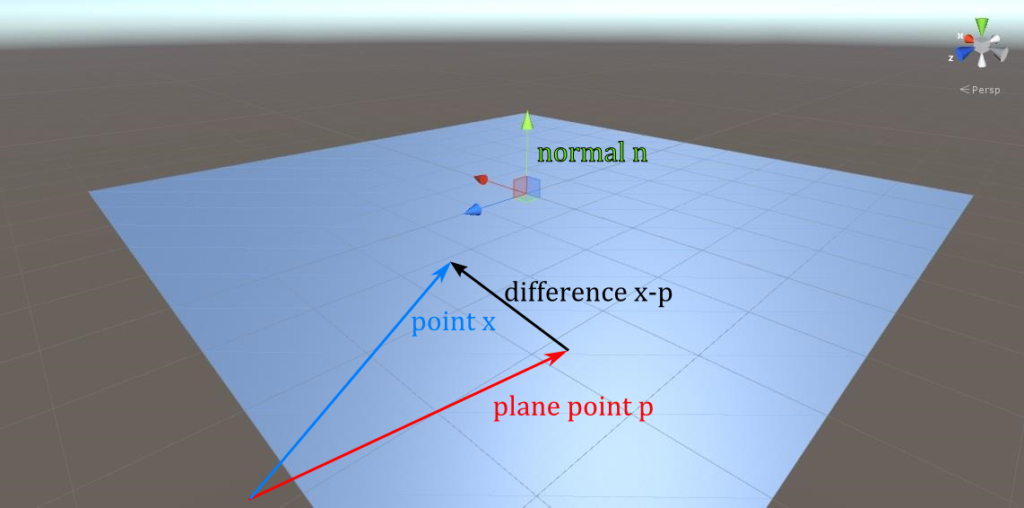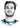# Howto Raytracer: Ray / Plane Intersection Theory

Categories:

In this tutorial I will derive how to calculate the intersection of a ray and a plane.

As already stated in my ray / sphere intersection howto, a ray $$r(t)$$ can be represented by a point on the ray $$e$$ and the ray’s direction $$d$$: $$r(t)=e + t d$$. The set $$R$$ of all points on the ray is then given by: $$R = {r(t) \mid t \in \mathbb{R}}$$

Similarly, a plane $$P$$ can be represented by a point on the plane $$p$$ and by its normal $$n$$. So how do we characterize the points on a plane? The main insight is that given any two points $$a,b$$ on the plane, the vector from $$a$$ to $$b$$, i.e. $$b-a$$, lies itself inside the plane and is thus by definition of the plane’s normal $$n$$ perpendicular to it. To check for perpendicularity, we check if the dot product $$(b-a)\cdot n$$ is $$0$$. The set of the plane’s points $$x$$ is then given by $$P = {(x-p) \cdot n = 0 \mid x \in \mathbb{R}^3}$$To find the intersection of the ray and the plane now, we have to find the points that are in both sets. So we check if a point $$r(t)$$ on the ray also fullfills the plane equation:

\begin{aligned} (r(t) - p) \cdot n = 0 \\ (e + t d - p) \cdot n = 0 \\ e \cdot n + t d \cdot n - p \cdot n = 0 \\ t = \frac{p \cdot n - e \cdot n }{d \cdot n} \\ t = \frac{(p - e) \cdot n }{d \cdot n} \end{aligned}

So we just calculate $$t$$, plug it back in the ray’s equation $$r(t)$$ and end up with the hit point, unless the denominator $$d \cdot n$$ is $$0$$ in which case there is no intersection. Geometrically this corresponds to the ray and the plane being parallel.

Here’s some sample code that implements this collision test:

    public override RayTracer.HitInfo Intersect(Ray ray)
{
RayTracer.HitInfo info = new RayTracer.HitInfo();

Vector3 d = ray.direction;
float denominator = Vector3.Dot(d, normal);

if (Mathf.Abs(denominator) < Mathf.Epsilon) return info;      // direction and plane parallel, no intersection

float t = Vector3.Dot(center - ray.origin, normal) / denominator;
if (t < 0) return info;    // plane behind ray's origin

info.time = t;
info.hitPoint = ray.GetPoint(t);
info.normal = normal;
return info;
}

All in all, ray / plane intersection is rather easy if you ‘re working with inifinitely long planes. If your planes have a width and height, it gets drastically more complicated using just a normal to represent a plane, because this representation is ambiguous to rotations around the normal. However for planes with finite width/height this orientation is important and you would be better suited representing the plane by the (orthogonal) vectors that span it.

# Hi, I'm Christoph Michel 👋I'm a , , and .

I mostly do and help small to mid-sized teams from all over the world 🌎 on both short and long-term projects on an independent contractor basis.

I strive for efficiency ⏱️ and therefore track many aspects of my life.